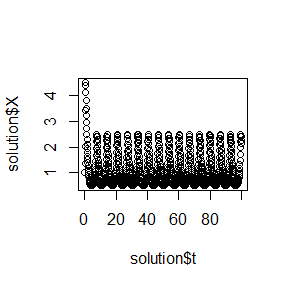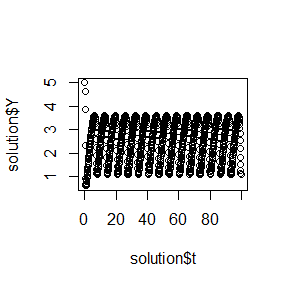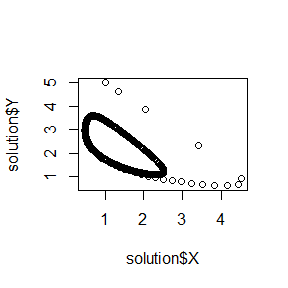Chemical Reaction

Build the Reaction class with a RK4 solver

library(rODE)
# This code can also be found in the examples folder under this name:
# Reaction.R

# /**
# * Reaction models an autocatalytic oscillating chemical reaction
# * (Brusselator model) by implementing the ODE interface.
# * @author Wolfgang Christian
# * @version 1.0
# * Converted to R by: Alfonso R. Reyes
#
# */

setClass("Reaction", slots = c(
k1 = "numeric",
k2 = "numeric",
k3 = "numeric",
k4 = "numeric",
A  = "numeric",
B  = "numeric",
state     = "numeric",
odeSolver = "RK4"
),
prototype = prototype(
k1 = 1.0, k2 = 2.5, k3 = 1.0, k4 = 1.0,      # reaction rates
A = 1, B = 1,                                # reactant concenterations
state = c(0, 0, 0)      # X, Y, t
),
contains = c("ODE")
)

setMethod("getState", "Reaction", function(object) {
object@state
})

setMethod("getRate", "Reaction", function(object, state, ...) {
xxy <- state * state *state
object@rate <- object@k1 * object@A - object@k2 * object@B * state +
object@k3 * xxy - object@k4 * state                          # X rate
object@rate <- object@k2 * object@B * state - object@k3 * xxy # Y rate
object@rate <- 1                         # rate of change of time, dt/dt
object@rate                                 # time derivative
})

# constructor
Reaction <- function(initialConditions) {
.Reaction <- new("Reaction")
.Reaction@state <- initialConditions
.Reaction
}
##  "getState"
##  "getRate"

Run the application ReactionApp

# +++++++++++++++++++++++++++++++++++++++++++++++++++ application: ReactionApp.R
# ReactionApp solves an autocatalytic oscillating chemical
# reaction (Brusselator model) using
# a fourth-order Runge-Kutta algorithm.

ReactionApp <- function(verbose = FALSE) {
X <- 1; Y <- 5;
dt <- 0.1
reaction <- Reaction(c(X, Y, 0))
solver  <- RK4(reaction)

rowvec  <- vector("list")
i <- 1
while (getState(reaction) < 100) {             # stop at t = 100
rowvec[[i]] <- list(t = getState(reaction),
X = getState(reaction),
Y = getState(reaction))
solver   <- step(solver)
reaction <- getODE(solver)
i <-  i + 1
}
DT <- data.table::rbindlist(rowvec)
return(DT)
}

solution <- ReactionApp()
plot(solution$t, solution$X)plot(solution$t, solution$Y)plot(solution$X, solution$Y)